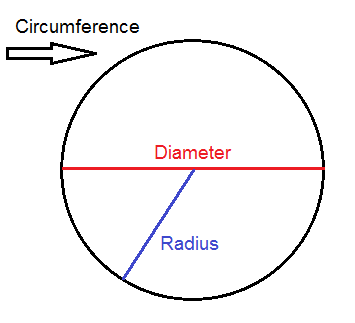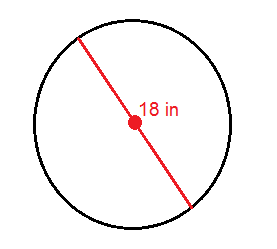# Calculating the circumference of a circle

The distance around a rectangle or a square is as you might remember called the perimeter. The distance around a circle on the other hand is called the circumference (c).A line that is drawn straight through the midpoint of a circle and that has its end points on the circle border is called the diameter (d)

Half of the diameter, or the distance from the midpoint to the circle border, is called the radius of the circle (r).

The circumference of a circle is found using this formula:

$\begin{matrix} C=\pi \cdot d\\or\\ \, C=2\pi \cdot r \end{matrix}$

Example

Find the circumference of the circle:$C=\pi \cdot d$

$C=\pi \cdot 18$

$C\approx 56.5\, in$

## Video lesson

Find the radius of the circle